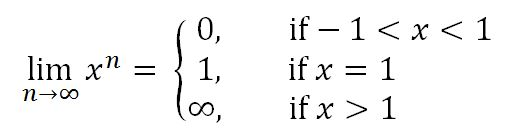# Introduction to sequences

### Introduction to sequences

In this lesson, we will talk about what sequences are and how to formally write them. Then we will learn how to write the terms out of the sequences when given the general term. We will also learn how to write the general term when given a sequence. After learning the notations of sequences, we will take a look at the limits of sequences. Then we will take a look at some definitions and properties which will help us take the limits of complicating sequences. These theorems include the squeeze theorem, absolute value sequences, and geometric sequences.

#### Lessons

Note:
1. If a sequence has the limit $L$, then we can say that:

$\lim$n →$\infty$ $a$$n$$=L$

If the limit is finite, then it is convergent. Otherwise, it is divergent.

2. If the limit of the sequences {$a_n$} and {$b_n$} are finite and $c$ is constant, then we can say that

i) $\lim$n →$\infty$ $(a_n+b_n)=\lim$n →$\infty$ $a_n+$$\lim$n →$\infty$ $b_n$.
ii) $\lim$n →$\infty$ $(a_n-b_n)=\lim$n →$\infty$ $a_n-$$\lim$n →$\infty$ $b_n$.
iii) $\lim$n →$\infty$ $ca_n=c$ $\lim$n →$\infty$ $a_n$.
iv) $\lim$n →$\infty$$(a_nb_n)=$ $\lim$n →$\infty$$a_n*$ $\lim$n →$\infty$ $b_n$.
v) $\lim$n →$\infty$ $[a_n$$\div$$b_n]$ $=\lim$n →$\infty$$a_n$$\div$ $\lim$n →$\infty$$b_n$$,$$b_n\neq0$.

3. If $a_n\leq c_n\leq b_n$ and $\lim$n →$\infty$ $a_n=$ $\lim$n →$\infty$ $b_n=L$, then $\lim$n →$\infty$ $c_n=L$.

4.if $\lim$n →$\infty$ $|a_n|=0$, then $\lim$n →$\infty$ $a_n=0$ as well.

5. We say that:Where the sequence {$x^n$} is convergent for -1< $x \leq$ 1, and divergent if $x$ > 1.
• Introduction
Overview:
a)
Notation of Sequences

b)
Definitions and theorems of Sequences

• 1.
Finding the terms of a sequence

Find the first five terms of the following sequences.
a)
$a_n=3(-1)^n$

b)
$a_n$= $\frac{n+1}{\sqrt{n+1}}$

c)
{$cos(\frac{n\pi}{2})$}

• 2.
Finding the formula for a sequence

Find the formula for the general term $a_n$ for the following sequences
a)
{$\frac{1}{2}, \frac{1}{3}, \frac{1}{4}, \frac{1}{5}, ...$}

b)
{$\frac{1}{2}, \frac{2}{5}, \frac{3}{8}, \frac{4}{11}, ...$}

c)
{-1, 4, -9, 16, ... }

• 3.
Convergence and divergence of sequences

Evaluate the limits and determine if the following limits are converging or diverging.
a)
$\lim$n →$\infty$ $\frac{(-1)^n}{n^2}$

b)
$\lim$n →$\infty$ $6(\frac{1}{2})^n$

c)
$\lim$n →$\infty$ $\frac{n^3+n+1}{n^2+1}$